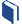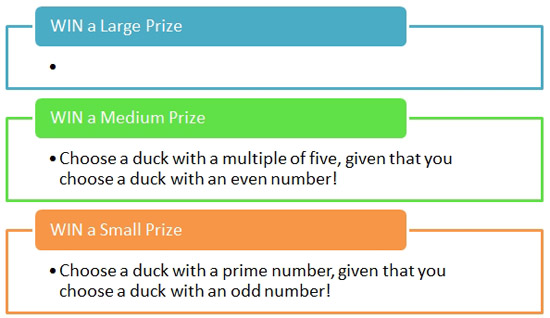# Prize Winner, Part 1

###Resources for this lesson:

> Glossary> Calculator Resources> Teacher Resources: Instructional NotesKhalid: Ok, so what do we want to be the rule for winning a medium prize?Allyson: How about "Choose a multiple of five, given that you choose an even number"?

The group agrees. As Khalid enters this into the Rules of the Game sign, the others find out what the probability is for winning a medium prize.In order to find P(choosing a multiple of five given that you choose an even number), first consider what numbers we are limited to. The gamer must choose an even number.

• List the even numbers.

So, the gamer is limited to fifteen numbers. From these fifteen (even) numbers, the gamer must choose a multiple of five.

• From the list of the even numbers, identify the numbers that are a multiple of five.

Thus, the probability of choosing a multiple of five given that you choose an even number is 3 out of 15, which can be written as $\frac{3}{15}=\frac{1}{5}$.